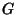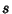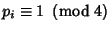## Conjugacy Class

A complete set of mutually conjugate Group elements. Each element in a Group belongs to exactly one class, and the identity () element is always in its own class. The Orders of all classes must be integral Factors of the Order of the Group. From the last two statements, a Group of Prime order has one class for each element. More generally, in an Abelian Group, each element is in a conjugacy class by itself. Two operations belong to the same class when one may be replaced by the other in a new Coordinate System which is accessible by a symmetry operation (Cotton 1990, p. 52). These sets correspond directly to the sets of equivalent operation.

Letbe a Finite Group of Order, and letbe the number of conjugacy classes of. Ifis Odd, then(Burnside 1955, p. 295). Furthermore, if every PrimeDividingsatisfies, then(Burnside 1955, p. 320). Poonen (1995) showed that if every PrimeDividingsatisfiesfor, thenReferences

Burnside, W. Theory of Groups of Finite Order, 2nd ed. New York: Dover, 1955.

Cotton, F. A. Chemical Applications of Group Theory, 3rd ed. New York: Wiley, 1990.

Poonen, B. Congruences Relating the Order of a Group to the Number of Conjugacy Classes.'' Amer. Math. Monthly 102, 440-442, 1995.